# Back to Riemann, Part 11

The entire point to this discussion is, “How do we estimate the distribution of the prime numbers?”

At one stage, I’d gotten into the idea of the prime counting functions, psi(x) and pi(x). pi(x) physically counts the primes less than x, and is approximated by pi(x) = x/ln(x). And psi(x) is a weighted summation of the powers of the primes. psi(x) = T2*ln(2) + T3*ln(3) + T5*ln(5) + T7*ln(7)… I.e. – psi(10) = 3*ln(2) + 2*ln(3) + ln(5) + ln(7) = 7.830 (there are three powers of 2 – 2, 4, 8; two powers of 3 – 3, 9; and one each of 5 and 7). As x gets larger, psi(x) approaches x, and pi(x) approaches x/ln(x). Therefore, psi(x) approaches pi(x)*ln(x).

der Veen and de Craats give a more accurate equation, called the “Explicit Formula for psi(x),” without going into the details for how Riemann derived it, or how von-Mangoldt simplified it. The details can be found in the wiki article.Although psi(x) gets close to x as x goes to infinity, there is some error that can be accounted for through a correction factor. In this case, ln(2*pi) just shifts the diagonal line y = x down by 1.83787… If x is greater than 1,000, then this term can effectively be ignored.

The third term is a summation of all the zeros of the zeta function, both the trivial and non-trivial ones. If we separate them out, as follows,as the summation of all the trivial zeros (x raised to the trivial zero divided by that zero; i.e. x^(-2)/(-2) + x^(-4)/(-4) + x^(-6)/(-6)…), and the summation of all the non-trivial zeros.

If we go back to the series expansion for ln(1 + y),
ln(1 + y) = y – (y^2)/2 + (y^3)/3 – (y^4)/4 + (y^5)/5…

plugging in y = -1/x^2 and multiplying both sides by 1/2 gives:
1/2 * ln(1  – 1/x^2) = 1/2 * ( -1/(x^2) – 1/(2*x^4) – 1/(3*x^6) – 1/(4x^8)…
= (x^-2)/(-2) + (x^-4)/(-4) + (x^-6)/(-6) + (x^8)/(-8)…

recognizing that this last form is the summation of the trivial zeros,
the trivial summation can be simplified as:

1/2 * ln(1 – 1/x^2).

If x = 50, then x^2 = 2,500, and 1 – 1/2500 = 0.9996.
-1/2*ln(0.9996) = 0.0002

Which is very small, and goes to 0 fast as x gets bigger. Therefore, the ln(2 * pi) and the 1/2 * ln(1 – 1/x^2) terms can be ignored as x goes to infinity (they total less than 2). They are needed to get the exact value of psi(x), but it’s pretty obvious that the main contribution to psi(x) is from the non-trivial zeros.

-1.837877… + 0.0002 = -1.837677…
If x = 50, and psi(x) approximates x, then the contribution from -ln(2*pi) – 1/2*ln(1 – 1/x^2) is only 3.67%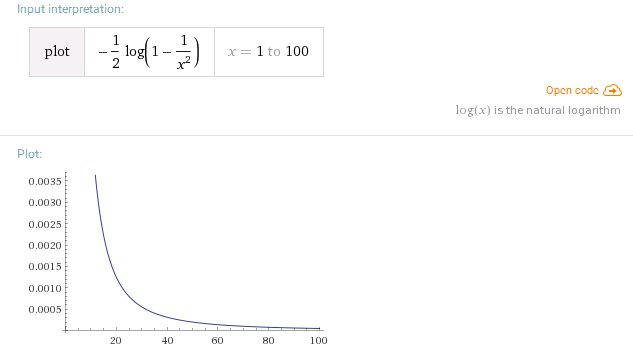(The sum of the trivial zeros goes to zero pretty fast.)

Since what we’re really interested in is the distribution of prime numbers over 100 digits long, we can ignore the correction factors and just focus on the term with the non-trivial zeros.

The next step is referred to as pairing up the non-trivial zeros. Because the explicit formula is a sum of ALL the non-trivial zeros, we need to look at the ones in the negative half of the complex plane (b*i < 0) as well as those in the positive half (b*i > 0). We can do this by pairing them up and looking at the angle they form. (Note, I’m using mirror() here to represent the mirror value of a complex number.)

If u = v + iw is a zero, then so is mirror(u) = v – iwThe summation of the non-trivial zeros is in the form of -(x^p)/p, so adding our zero “u” and mirror(u) is going to look like:

eq. 1) -(x^u)/u – (x^mirror(u))/mirror(u) = -(2/|u|) * (x^v) * cos(w*ln(x) – a)

And we do this for all of the non-trivial zeros.
(|u| is the absolute value of u.)

Now, the thing that’s interesting about this pairing is it’s independent of the opposite mirror: 1 – v. That is, while we’re pairing v + bw up with v – bw, we need to make a separate pairing of 1 – v + wi with 1 – v – wi.

Why is this important? Because it gives us 4 zeros, not 2.
But, Riemann’s hypothesis says that all of the zeros are on the critical line, so we should only have 2 matching zeros, not 4, and v would = 1/2 in all cases. This simplifies the math, as well as minimizing the impact of the non-trivial zeros on the explicit formula for calculating psi(x). If the hypothesis fails, this double pair is going to have twice as much weight as the zeros that are on the critical line.

Ok, so what do these paired non-trivial zeros look like?
The easiest way to find out is to break eq. 1 down into individual components.
u = v + iw
|u| = sqrt(v^2 + w^2)
a = atan(2*w/(2*v)) = atan(2*w) (for v = 1/2)

Then, what are v and w?
They’re the zeros for the zeta function. I’ve already stated that all the zeros that have been found so far lie on the critical line, so v = 1/2. I’ve calculated the stats for the first 10 zeros in Excel. As the zeros get bigger, “a” gets closer to pi/2 = 1.570796Then, what I did was to set up a spreadsheet where x runs from 1 to 100 in 0.2 increments in the F column. vk is in the A column, wk in the B column, |uk| is in the C column, and ak (atan(2*wk)) is in the D column.
G column is then the paired non-trivial zero equation:
=(2/\$C\$2)*POWER(\$F2,\$A\$2)*COS(\$B\$2*LN(\$F2) – \$D\$2)

And, for the first non-trivial zero (0.5 + i*14.13457), the graph for x = 1 to 100 is:The 10th zero is at 0.5 + 49.77383, and the graph is:Summing the results of the first 10 zeros and subtracting from x, for x = 1 to 100, gives the following graph, which is plotted along with psi(x). Remember that psi(x) counts the powers of each prime (i.e. -> 2, 4, 8, 16, 32, 64) from 1 to x, and sums the weighted primes as:
psi(x) = T2*ln(2) + T3*ln(3) + T5*ln(5) + T7*ln(7)
where, if x=10, T2=3, T3=2, T5=1 and T7=1This is just for the first 10 zeros. Adding all the other known zeros to infinity creates a step function that is a near-perfect match for psi(x).

Where does this leave us? Well, we know that the Riemann zeta function can be extended to the entire complex plane, with the exception of x = 1. We know that the zeros for the zeta function lie in the critical strip 0 < x < 1, that the zeros can be paired up in the explicit formula for psi(x), and that the known non-trivial zeros lie on the critical line x = 1/2, which is covered by the eta(x) function. If we plug the known zeros into the explicit formula and subtract this from x we get psi(x), which is a prime counting function that physically does count the prime powers and is then a weighted sum of those powers from 2 to x.

Actually, there are two things left I want to deal with. Creating an Excel file for confirming the first few known zeros, and addressing why having a zero that is not on the critical line messes everything up.

# Back to Riemann, Part 10

Ok, back to the der Veen and de Craats version of the Riemann functional equation.

z( -x ) = ((-2 * x!) / (2*pi)^(x+1)) * sin(pi*x/2) * z(x + 1)

What this states is that if you have the zeta(x) value for any complex x (x = a + bi) then you can get zeta(x + 1), and from there apply the functional equation to define zeta(-x).

This extends the zeta function to the left hand side of the complex plane (a < 0). Combining the functional equation with eta(x) and the original version of zeta(x) for x>1, we now have complete coverage of the complex plane everywhere except for the pole point x = a = 1.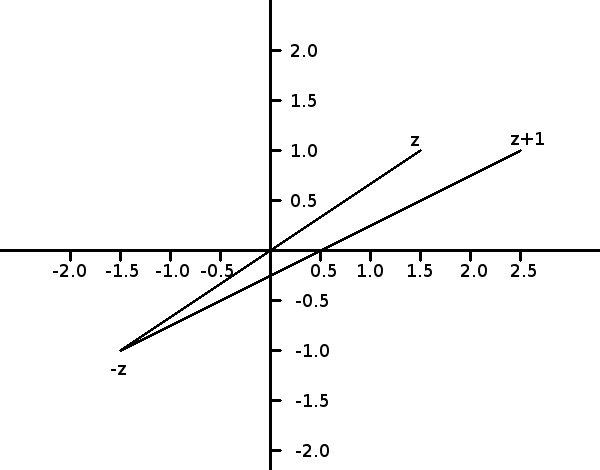One interesting feature here is that the mirror relationship between -z and z + 1 gives a center of rotation at x = 0.5.
c = B + (A – B)/2 = -z + (z + 1 – (-z))/2
c = -z + (2z + 1)/2
c = -z + 2z/2 + 1/2
c = 0.5, for any z.

I’ve mentioned before that the zeta(x) function converges to 1 for large x, meaning that there are no zeros for x>1.

And, the functional equation relates -z to z + 1, so again, there are no zeroes for x < 0, except for the trivial zeroes at x = -2, -4, -6, etc, because of the sin() component. (If there were any other zeroes to the left of x = 0, then there would have to be matching zeroes for x > 1, and there aren’t.)That just leaves what is called the “critical strip,” for x = a + bi, 0 <= a < 1, which is covered by the eta(x) function.

Given the nature of the complex plane, if we know the value for x = a + bi, then we also have the values for a – bi, -a + bi and -a – bi. Which means that if zeta(a + bi) = 0, then zeta(a – bi) = zeta(-a + bi) = zeta(-a – bi) = 0.

That is, if there is a zero within the critical strip, it will have 3 more matching zeroes to go with it.

What Riemann did with his hypothesis was minimize the number of possible zeroes, by saying that all of the non-trivial zeroes lie on the critical line x = 0.5. Or, instead of having 4 zeroes for any given a + bi combination, there are only 2 (a + bi and a – bi).

So far, no one has been able to prove OR disprove this statement. Billions of zeroes have been found by using computers, and they’re all on the critical line. But, that’s not a proof. If even just one zero exists that is not on the critical line, then the entire hypothesis collapses, as do all the other theorems that are based on it.

Ok, so that’s the basic concept of the zeta function. We have the regular version,
z = 1 + 1/2^x + 1/3^x + 1/4^x…

For x > 1 across the complex plane.

We have the eta(x) function,
eta(x) = 1 – 1/2^x + 1/3^x – 1/4^x…

followed by,
zeta(x) = eta(x) / (1 – 2^(1-x))

for 0 < x < 1 along the complex plane.

And Riemann’s functional equation above, for x < 0.

If we want to find the non-trivial zeroes, we need to use the eta(x) plus the conversion formula.

In order to follow the examples in the book, I’ve been playing around with Wolfram Alpha. It’s a pretty decent online math graphing tool, but there are some inconsistencies between the version online now and what der Veen and de Craats apparently used (dVdC say that the “|” character is used to calculate absolute values, i.e. “plot |x| for x = -10 to 10”. But that doesn’t work for me. I have to use “plot abs(x) x = -10 to 10). Most of the inconsistencies are minor, but it still takes time to figure them out.

The main thing is that because Wolfram Alpha already has the zeta function implemented, it’s very easy to see right away what it looks like near the origin. If we plot zeta(x) for (-30 -30i) to (30 + 30i), we get two graphs, one of the real component of the results and the other for the imaginary component.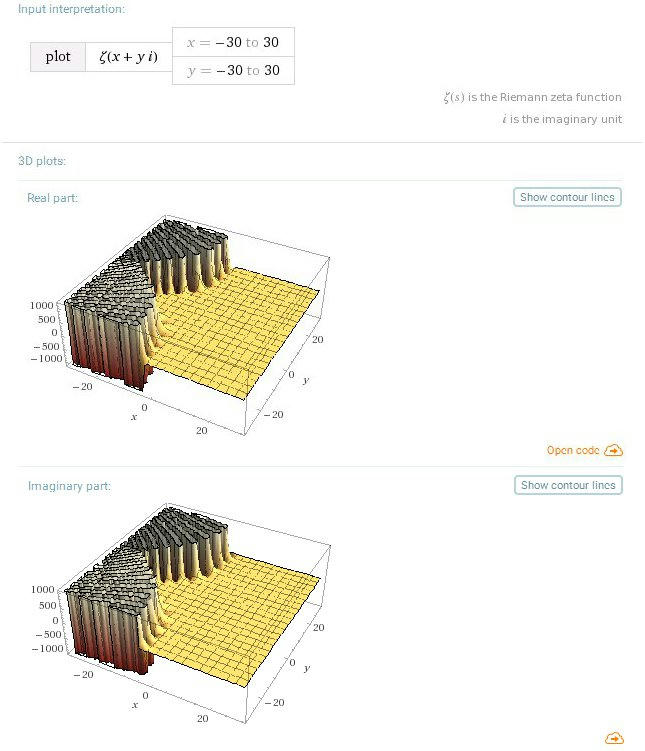(plot zeta[x + y*I] x = -30 to 30 y = -30 to 30)

Note that the results for both components get clipped at z = 1000. The important part of the complex plane is that strip between 0 and 1, where the results weave around z = 0, but that’s getting swamped out.

Plotting the absolute value of the above formula, we get the combined magnitudes of the real and imaginary components, but clipping is now occurring at 10^9. No zeroes visible from this altitude.(plot abs(zeta[x + y*I]) x = -30 to 30 y = -30 to 30)

The next step is to concentrate only on the critical strip, and to try to include at least one of the zeroes. Remember, the zeroes themselves are on the critical line, x = 0.5.(plot zeta[x + y*I] x = 0 to 1 y = -30 to 30)

And the absolute value of the plot as well. Note the pole at x = 1.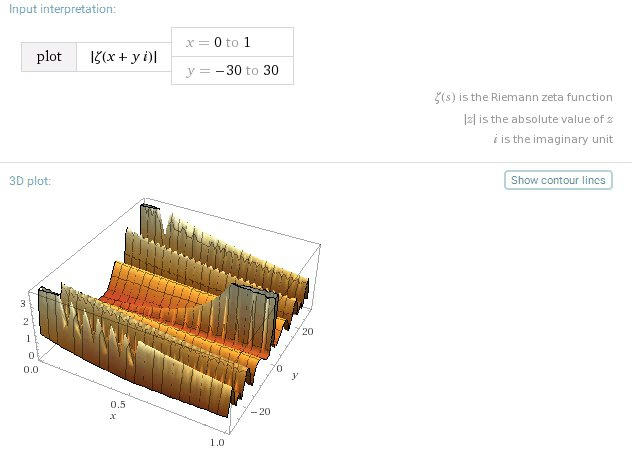(plot abs(zeta[x + y*I]) x = 0 to 1 y = -30 to 30)

We can try plotting just the critical line, and to find the zeroes we need to see where both the real and imaginary components cross the x-axis at the same time.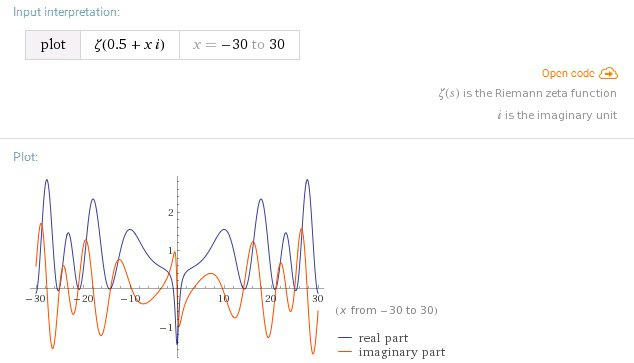(plot zeta[0.5 + y*I] x = -30 to 30)

Or, we can look at the absolute value of the graph, where the zeroes are the points where the line touches down on the axis. Regardless, the mirror nature of the complex plane is visible here. So, we only need to find the zeroes for y > 0 and we automatically get the matching zeroes for y < 0 at the same time.(plot abs(zeta [1/2 + x*I]) x = -30 to 30)

This just leaves the graphs of the trivial zeroes.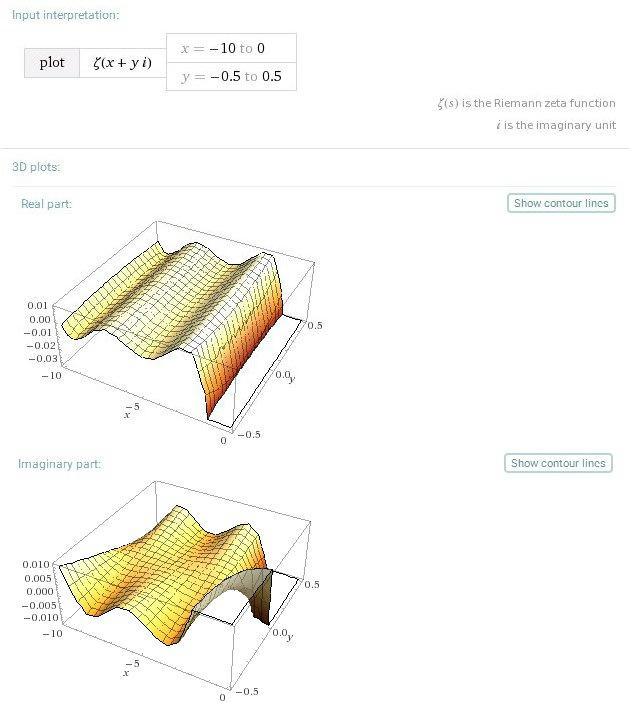(plot zeta [x + y*I] x = -30 to 0 y = -0.5 to 0.5)(plot abs(zeta [x + y*I]) x = -30 to 0 y = -0.5 to 0.5)

As x goes more negative, the results get much bigger faster, swamping the y-axis crossings. So, I’m only showing the first few trivial zeroes, for x = -10 to 0.(plot zeta [x] x = -10 to 0)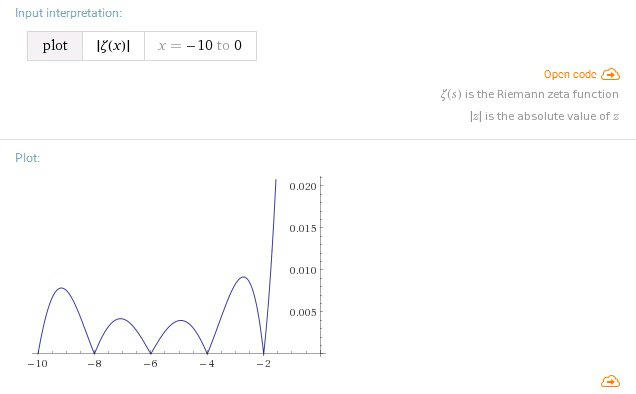(plot abs(zeta [x]) x = -10 to 0)

While I’m at it, I might as well include a little of the gamma function. I’ll just use the part closer to the origin to show the best detail.(plot gamma[x + I*y ] x = -10 to 0 y = -10 to 10)(plot abs(gamma[x + I*y ]) x = -10 to 0 y = -10 to 10)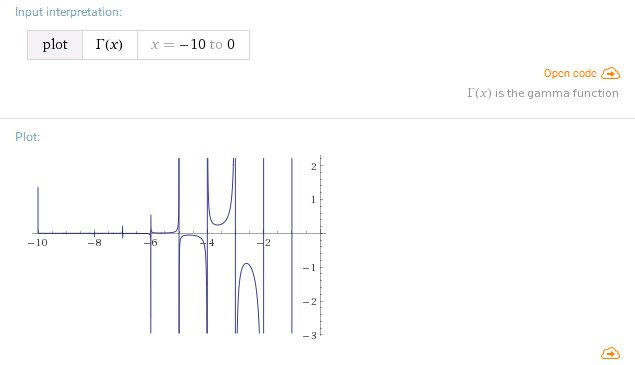(plot gamma[x ] x = -10 to 0. Note the zero crossings at -2, -4, -6, etc.)

Next time: Tying the non-trivial zeroes to the Prime Counting Function

# Pi*r^2

Happy Pi*r^2 Day.

# Back to Riemann, Part 9

Riemann was interested in understanding heat flow in a wire. As he was analyzing this, he developed a series of tools that could also be applied to the prime number distribution system.

What he came up with is the Riemann Functional Equation, which, according to der Veen and de Craats, is explained in more detail in chapter 9 of Jeffrey Stopple’s book “A Primer of Analytic Number Theory, From Pythagoras to Riemann.” This functional equation uses another mirror property of complex numbers to relate values to the left of the y-axis (x < 0) to those on the right side (x > 0), specifically as it applies to the zeta function.

z( -x ) = ((-2 * x!) / (2*pi)^(x+1)) * sin(pi*x/2) * z(x + 1)
(from the der Veen and de Craats book)

or,(Image from the wiki article.)

That is, if you know zeta(x + 1) where x >= 0, then you can use this conversion function to get z( -x ).
Although both zeta(x) and eta(x) go to infinity for x < 0, using the functional equation extends the domain of zeta(x) to the entire complex plane, except for x=1, which still has a pole.

I’ll get to the idea of finding factorials of complex numbers in a minute.
Right now, if you look at the functional equation closely, you’ll see it has 4 parts:

-2 * x!
(2*pi)^(x+1)
sin(pi*x/2)
zeta(x+1)

We already know that zeta(x+1) doesn’t have zeroes for x > 1 across the complex plane, and that it converges to 1 for large x.
x! doesn’t have zeroes.
And (2*pi)^(x+1) doesn’t have zeroes.

However, sin(pi * x / 2) DOES go to 0 for integer multiples of pi.
0 = sin(0) = sin(pi) = sin(2*pi) = sin(3*pi) = sin(4*pi)…

And in the functional equation, that happens when x = -2, -4, -6, -8, etc.

These are the trivial zeroes, and they occur at all negative even integers of x.

Remember, the Riemann hypothesis says that the non-trivial zeroes for the zeta function are on the critical line, x=1/2.
This implies that there are trivial zeroes that don’t contribute to the hypothesis.

And we now know where the trivial zeroes come from:
the sin(pi*x/2) component.

Ok, factorials.

Traditionally, factorials are written as x!, which represents the product of all integers from 1 to x.
Example:
1! = 1
2! = 2
3! = 6
4! = 24
5! = 120
6! = 720

If we treat this pattern as a continuous line through all of the data points, it’s going to look something like this: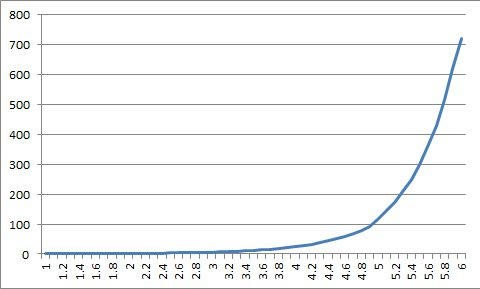Meaning that technically we can have 3.3!, or 5.921!
It just means that the result is going to fall somewhere along the this line.

Now, trying to do something like this with imaginary numbers would be a little trickier, but it’s still something that can be visualized.
i! = i
2i! = i * 2i = -2
3i! = -2 * 3i = -6i
4i! = -6i * 4i = 24
5i! = 24 * 5i = 120i

which represents a rapidly growing spiral.
3.3i! would then be somewhere along that spiral between -6i and 24, which could be found with a variation of cos(x) + i*sin(x).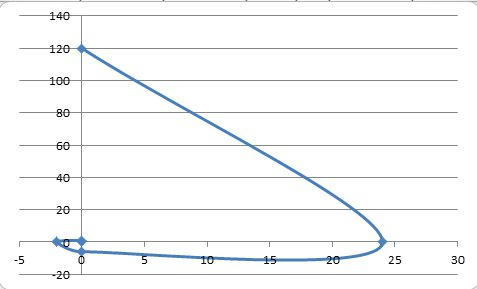(Not really a proper spiral, but I think you get the point.)

Which is all really irrelevant when you mix the two, and try to get something like
(-6 + 4i)!

This is where the gamma function comes in. The gamma function is Riemann’s approach to complex factorials.

It’s also where I ran into a complete roadblock the first time I tried graphing the zeta function in my earlier blog series. The wiki entry was too difficult for me to follow  at that time, and right now, der Veen and de Craats completely whiff out by referring to the wiki article.

However, there’s more than one way to approximate the gamma function, and the wiki article for the Lanczos approximation does have a code example in Python that I might try to steal from.

Briefly, the Lanczos approach uses a set of coefficients that can be pre-calculated and plugged into a table, then the gamma equation itself can be calculated as:

where “g” is an arbitrary constant with the real part > 1/2, and Ag(z) is:

If you pre-choose z, Ag(z) is just the sum:

Why go through all this work?

Well, Excel does have a gamma function built-in, but it’s only for real values of x, and doesn’t support complex values. Wolfram Alpha supports complex values for gamma, but it’s difficult to create the kind of animations I want to make.

On the other hand, the animations I’m most interested in are those for the zeta function in the critical strip (0 < x < 1), and that just requires the eta function, not the Riemann functional equation. So, this is “nice to know” material, but not essential. Then again, there’s a key part of the critical strip that I haven’t addressed yet that does depend on knowing what the functional equation is, and I’ll get to that next time.

The main reason for going through all this work, though, is to be able to prove to myself that I can write up the zeta function for the entire complex plane in one format or another. If not in Excel, then in VBScript (which I can then use to load values into Excel for graphing purposes).

# Back to Riemann, Part 8

Ok, yes, finally, extending the domain for the zeta function to the complex plane.

Up to this point, the conventional form of the zeta function:
z(x) = 1 + 1/2^x + 1/3^x + 1/4^x…

only converged to some constant if x was a real number greater than 1.
By introducing the eta function, we were able to extend the domain to real numbers greater than or equal to 0, but not including 1, which has a pole.

This is the same as saying that the zeta(x) function works for x = a + bi, where b = 0, and x > 1. And the eta(x) function works for x = a + bi, where b = 0, and 0 <= x < 1.

But, say we use the natural logarithm ln(x), instead of the current exponent form.
We know from part 5 that,
2 = e^ln(2)
and, from the rule of exponents that
2^z = e^(z*ln(2))

If z = a + bi,
2^(a + bi) = (2^a) * (2^bi) = (2^a) * e^(bi * ln(2))

But,
e^bi = cos(b) + i*sin(b)

So,
(2^a) * e^(bi * ln(2)) = (2^a) * (cos(b * ln(2)) + i*sin(b * ln(2)))

The reciprocal of 2^(a+bi) = 1/(2^(a + bi)) = 2^(-a -bi)

So, if we look at the above zeta function, the second term is 1/(2^x),
which we now know is:
(1/(2^a)) * (cos(b * ln(2)) – i*sin(b * ln(2)))

The third term is 1/3^x, so that’s
(1/(3^a)) * (cos(b * ln(3)) – i*sin(b * ln(3)))

And we just keep on doing this for every other term in the zeta function.
This is how we extend the domain for the zeta(x) function to the complex plane for all real x > 1.

There are two important points to note here.
First, the complex plane is a mirror around the x-axis. That is, if x1 = 5 + 3i,
then the mirror image point is x2 = 5 – 3i.

1/2^x1 = 1/2^(5 + 3i) = (1/2^5) * (cos(3 * ln(2)) – sin(3 * ln(2))
1/2^x2 = 1/2^(5 – 3i) = (1/2^5) * (cos(3 * ln(2)) + sin(3 * ln(2))

Which is going to hold for all of the other terms in the series. I.e.,
1/3^x1 = 1/3^(5 + 3i) = (1/3^5) * (cos(3 * ln(3)) – sin(3 * ln(3))
1/3^x2 = 1/3^(5 – 3i) = (1/3^5) * (cos(3 * ln(3)) + sin(3 * ln(3))

Because e^i involves rotation around the origin, the rotations generated by each term for x = 5 + 3i are going to be mirrored on the other side of the x-axis for x = 5 – 3i.
That is, zeta(5 + 3i) and zeta(5 – 3i) will also be mirror images of each other.
In short, we only need to calculate zeta(5 + 3i) and we’ll know what z(5 – 3i) is, too.

Second, the eta function.
The eta function is what let us extend the zeta domain to x >= 0.
It also has a complex plane version, and the conversion formula is
zeta(x) = eta(x) / (1 – 2^(1-x))

And, eta(x) was a modified version of the zeta function that converges faster:
eta(x) = 1 – 1/2^x + 1/3^x – 1/4^x + 1/5^x – 1/6^x

To get the eta function we just need to flip the signs of every even cos()/sin() term in the zeta series, and the conversion formula uses 2^(1-x) instead of 2^x, which is the first term in the zeta series. Going from the zeta(x) series to the eta(x) series and back will be easy.

Technically, I could stop here.
The Riemann Hypothesis states that all of the non-trivial zeroes of the zeta function lie on the critical line, x = 1/2.
And, with the eta(x) function, which is valid from x = 0 to x < 1, whose domain has just been extended to include the entire complex plane in that range, I can now write a usable version of the zeta function in Excel to make animated graphs as I navigate around the complex plane (I think; I haven't proved this, yet).

The thing is, I still haven't covered what the trivial and non-trivial zeroes are, I haven't extended the domain of the zeta function to x < 0, and I haven't talked about why the critical strip only extends from x = 0 to x < 1.

So, I'm going to get into some of that next time.

# Site problems – edited

WordPress support tells me they’ve fixed the problem that was causing images on mediafire to not display. If you still notice any missing images (such as, there’s a caption but no photo to go with it) please comment on this post and let me know. Thanks.

# Hachette 3D Puzzle Series, vol. 1Hachette is one of two international publishers selling serialized kits and/or magazines in Japan, which include TV show DVDs, 3D printer kits, drone and robot kits, etc. Hachette actually had a similar 3D puzzles line about 8-9 years ago, which consisted of 50 puzzles that came out one every 2 weeks. I’d missed most of those, and only discovered the series just about the time that it ended. I could only get a couple of the backissue volumes from Kinokuniya bookstore, because all the earlier ones were sold out.(Game history page, this one for Mancala.)

The current line will only have 18 issues total. The first one is 499 yen (\$4.50), which is why I picked it up. The other ones will probably all be about 1,300 yen each. If you get the full subscription right now, you get your choice of one more puzzle plus a copy of Mancala, or the “casino” game (the one where you have a dice box with wooden counters with the numbers 1-9 on them. Rolling a specific number on the dice lets you flip the counter over.)

Each volume will come with a magazine. The first mag is twice as long as the regular ones because it’s advertising for the series. The regular magazines will be shorter and all follow the same pattern. First will be a section on the history of a specific game, then there will be several pages of paper logic puzzles, including magic squares, Sudoku, and elimination grid puzzles (the kind where you are given the names, occupations, and cities of 5 people, plus a list of clues linking them together.) There’s also a classical painting memory game (you look at the painting for a few seconds, then try to answer questions about it from someone else, such as “how many people in the painting?”, “what animal is in the picture?”, “where in the picture is the animal?”, etc. All of the pages are 3-hole punched, so you can store them in a binder.

Three of the issues are things in the same vein as the Rubik’s cube, where you have to scramble, then unscramble them. One of those looks kind of pretty, so I may get it just based on its looks. However, I normally don’t like those things. One toy is going to be the Tower of Hanoi, and the rest look to be 3D wood puzzles like the ones I’d gotten from capsule ball dispensers last year. In fact, the Hachette line has 3 identical duplications of the capsule ones I already have and another 2 are just fancier versions of the capsule puzzles. Overall, I’m only interested in 7 or 8 of the issues, not including #1, which I already have. The next one I want is #6, which won’t be hitting the shelves until sometime in May, I guess. On the other hand, the magazine cover shows 3 other puzzles that aren’t in the series, so I don’t know how they figure into all of this.Ok, the toy for vol. 1 is this assembly puzzle. All the pieces are the same, and they form a cube about 2.5″ to a side. The holder has a flat corner so you can display the finished puzzle on-end like a kind of trophy. But, the pieces slide around easily and if the holder gets bumped, you’re going to find yourself trying to pick every single piece up from off the floor. This is going to be like a jigsaw puzzle – lose one piece and the entire thing becomes worthless.As of this writing, I haven’t tried putting it back together. But, I’ve got a pretty good idea of what the trick is. When I get a free hour, I’ll sit down and see how well I do. As for the paper puzzles in the magazine, there are a couple I want to try, including the Sudoku one, and the elimination grid. If you want to cheat, the solutions for those are at the back of the same issue.